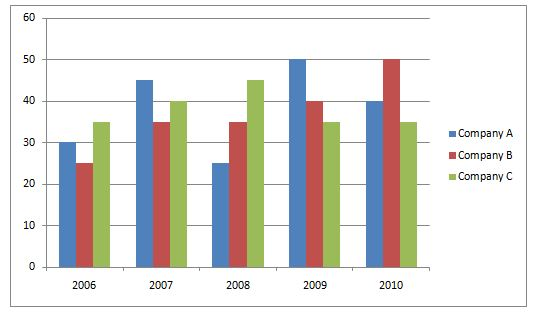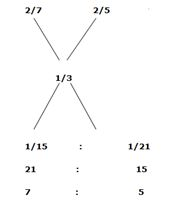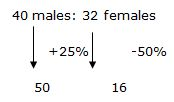# Crack SBI Clerk Prelims 2018 Sectional Full Test (Quantitative Aptitude) Day-5

Dear Readers, SBI Clerk (Junior Associates) 2018 Preliminary Examination is scheduled to be held on 23rd, 24th, and 30th of June 2018. In that case, aspirants need to speed up the preparation as there are only few days more.

To boost up your exam preparation, Our IBPS Guide team is providing a full length Sectional Tests for English, Quantitative Aptitude and Reasoning with detailed solutions to score more marks in the prelims exam. Make use of this opportunity and recommend to your friends to achieve a successful career in Banking.

You can also practice using our SBI Clerk Test Series pack prepared by our experienced professionals as per the latest exam pattern. We wish you a great success in all forthcoming exams. Click Here for SBI Clerk Practice Mock Test

#### Minimum Cut Off for this Quantitative Aptitude Section Test is: 22

[WpProQuiz 2501]

Click “Start Quiz” to attend these Questions and view Solutions

1). Praveen covers a distance by walking for 6 hours. While returning, his speed decreases by 2kmph and he takes 9 hours to cover the same distance. What was his speed while returning?
A. 2 kmph
B. 5 kmph
C. 4 kmph
D. 7 kmph

E. None

2) The average age of a group of persons going for tour to Srinagar is 22 years. 25 new persons with an average age of 10 years join the group and their average age becomes 12 years. The number of persons initially going for tour is?
A. 10
B. 8
C. 7
D. 5
E. 4

3) Ayisha goes to a supermarket and bought things worth rupees 60, out of which 40 paise went on sales tax. If the tax rate is 10 percent, then what was the cost of tax free items?
a) 54.60
b) 55.60
c) 56.60
d) 57.60
e) None of these

4) A toys manufacturing company declares 20 percent discount for whole sale buyers. A trader bought toys for rupees 4000 after getting the discount. Now he sells the toys at 15 percent profit on the original price of the company. What is the selling price of the toys?
a) 4750
b) 5740
c) 5750
d) 6750
e) None of these

5) One tap can fill a tank thrice as fast as another tap. If together the two taps can fill the tank in 12 minutes, then the faster tap alone will be able to fill the tank in

A) 30mins

B) 24mins

C) 12mins

D) 15mins

E) 16 mins

Direction (6-10): Study the following graph carefully and answer the given questions

The production of Cotton (in lakh tones) by three different companies A, B, C over the years.6) The average production for five years was maximum for which company?
a) A

b) B

c) C

d) A and C

e) C and B

7) In which year was the percentage of production of Company C to the production of Company B the maximum?

a) 2006

b) 2007

c) 2008

d) 2009

e) 2010

8) What is the percentage increase in the production of Company B from 2006 to 2009 ?
a) 45%

b) 50%

c) 60%

d) 65%

e) 70%

9) What is the difference between the production of Company C in 2008 and Company B in 2006?

a) 2,00,000 tons

b) 20,000 tons

c) 2,000 tons

d) 20,00,000 tons

e) 20 tons

10) Increase in percent of production of Company C from 2006 to 2007 is what percent of that increase percent of production of company B from 2009 to 2010 ?

a) 70.5%

b) 57.12%

c) 62.54%

d) 74.34%

e) 85.34%

Directions (11-15): In the following questions, two equations I and II are given. You have to solve both the equations.

a) If a > b

b) If a < b

c) If a ≥ b

d) If a ≤ b

e) If a = b or the relationship cannot be established

11) a² – 43a + 450 = 0
b² – 33b + 272 = 0

12) a² – 28a + 195 = 0
b² – 35b + 306 = 0

13) a² – 38a + 345 = 0
b² – 23b + 130 = 0

14) a² = 64
b² – 30b + 225 = 0

15) a² – 30a + 221 = 0
b² – 31b + 240 = 0

Directions (16-20): What approximate value should come in place of the x in the following questions? (You are expected to do approximations)

16) 342 – 831.94 ÷ 13 = x
A) 1303
B) 1345
C) 1388
D) 1457
E) 1092

17) √1765 + 1010 ÷ 14 – 64% of 250 = x
A) -46
B) -26
C) 32
D) -33
E) 39

18) (82)2 – (71)2 – (34)2 = x
A) 376
B) 527
C) 493
D) 525
E) 428
19) √6561 + 13 × 32 – 784 ÷ 14 = x2
A) 31
B) 39
C) 21
D) 29
E) 19

20) 32.06% of 150.38 * 53% of x = 7632.421

A) 156

B) 300

C) 200

D) 400

E) 120

Directions (21-25): find the missing number in the following number series?

21)  25, 100, ?, 1600, 6400

1. 400
2. 300
3. 360
4. 420
5. 320

22) 125, ?, 343, 512, 729, 1000

1. 216
2. 215
3. 256
4. 225
5. 226

23) 1 , 27 , 125 , 343 , ? , 1331

1. 730
2. 729
3. 512
4. 772
5. 289

24) 121, 144, 169, ?, 225

1. 180
2. 172
3. 186
4. 196
5. None of these

25) ?, 2116, 2209, 2304, 2401, 2500

A) 2124

B) 1972

C) 1521

D) 2025

E) 2024

26). Two trains run in the same direction at 50kmph and 104 kmph respectively. If the faster train crosses a man sitting in the slower train in 22 seconds, then the length of the faster train is

A) 330m

B) 350m

C) 325m

D) 305m

E) 400m

27). A man gets a Simple interest of Rs. 1000 on a certain principal at the rate of 5 p.c.p.a in 4 years. What compound interest will the man get on twice the principal in two years at the same rate?

A) Rs.1050

B) Rs.1005

C) Rs.1105

D) Rs.1012

E) Rs.1025

28). If the numerator increased by 200% and denominator increased by 150%, resultant fraction equals to 7/10. What is the original fraction?

A) 5/12

B) 7/12

C) 12/5

D) 18/23

E) 30/53

29). There are two vessels containing the mixture of milk and water. In the first vessel the water is 2/5rd of the mixture and in the second vessel water is 2/7 of the mixture. In what ratio these are required to mix to make mixture in which the ratio of water to milk is 1:2?

A) 4:3

B) 5:7

C) 5:2

D) 7:5

E) 3:5

30). A bag contains 4 red, 5 green and 6 violet balls. If 3 balls are drawn randomly, then what is the probability of all the balls are green?

1. 1/91
2. 3/91
3. 4/91
4. 2/91
5. 7/91

Directions (31-35) Study the following table carefully and answer the questions

Total no. of passengers in each coach are 80 on (Saturday). All passengers were present in each coach.

Table shows no. of passengers travelled in a particular day (Saturday)

 Coach no No. of child Male : Female B1 15 8:5 B2 12 9:8 B3 8 5:4 B4 10 10:4 B5 12 8:9 B6 16 6:10 B7 15 9:4

31). Find the total number of adults in B1, B2, B3?

A) 240

B) 278

C) 204

D) 205

E) 145

32). What is the ratio of males travelling in B1 to females in B3?

A) 5: 4

B) 4: 5

C) 2: 3

D) 2:5

E) 4:7

33). If male adults increased by 25% and females decreased by 50% in B3, then total adults in B3 are?

A) 55

B) 46

C) 66

D) 76

E) 85

34). What is the percentage of adult males to adult females in coach B3?

A) 125%

B) 25%

C) 33 1/3%

D) 75%

E) 150%

35). What is the ratio of adult passengers in B3 to B4?

A) 55:56

B) 35:36

C) 36:35

D) 76:77

E) 85:86

The speed of Praveen in return journey = x
6(x + 2) = 9x
=> 6x + 12 = 9x
=> 9x – 6x = 12
x = 4kmph

Initial number of persons = x
22x + 25 * 10 = 12(x + 25)
22x + 250 = 12x + 300
10x = 50
x = 5

Tax = 40/100 = (10/100)*T, T = 4
so cost of tax free items = 60 – 4 – 0.40 = 55.60

Let original price of toys be M
(80/100)*M = 4000, M = 5000
Now to gain 15% on the original price, the trader will sell the toys at (115/100)*5000 = 5750 rupees

(1/x)+(1/3x)=(1/12)

(3+1)/3x = 4/3x = 1/12

3x = 48

x=16 mins

Direction (6-10)

Company A = (30+45+25+50+40)/5 = 38

Company B = (25+35+35+40+50)/5 = 37

Company C = (35+40+45+35+35)/5 = 38

2006 = (35/25)*100 = 140%

2007 = (40/35)*100 = 114.29%

2008 = (45/35)*100 = 128.57%

2009 = (35/40)*100 = 87.5%

2010 = (35/50)*100 = 70%

In the year 2006 has maximum production of company C compared to the production of company B

Required percentage = [(40-25)/25]*100 = 60%

Required difference = [(45-25)*100000] = 20,00,000 tons

Production of Company C in 2006 = 35

Production of Company C in 2007 =40

Increase % = [(40-35)/35]*100 = 14.28%

Production of Company B in 2009 = 40

Production of Company B in 2010 = 50

Increase % = [(50-40)/40] = 25%

Required % = (14.28/25) * 100 = 57.12 %

Direction (11-15)

a² – 43a + 450 = 0

a2– 18a – 25a +450 =0

a(a-18) – 25( a-18) =0

(a-25) (a-18) =0
a = 25, 18
b² – 33b + 272 = 0

b2– 17b -16b +272 =0

b(b-17) -16 (b-17) =0

(b-16) (b -17) =0
b = 17, 16

a² – 28a + 195 = 0

a2-13a-15a +195 =0

a( a-13) -15 (a-15) =0

(a-13) (a-15) =0
a = 13, 15
b² – 35b + 306 = 0

b2-17b -18b +306 =0

b(b-17)-18 (b-17) =0

(b-17) (b-18) =0
b = 17, 18

a² – 38a + 345 = 0

a2-23a-15a+345 =0

a( a-23) – 15 (a-23) =0

(a-23) (a-15) =0
a = 23, 15
b² – 23b + 130 = 0

b2– 10b-13b+130=0

b(b-10) -13 (b-10) =0

(b-10) (b-13) =0
b = 10, 13

a² = 64
a = 8, – 8
b² – 30b + 225 = 0

b2-15b-15b+225 =0

b(b-15) – 15 (b-15) =0

(b-15) (b-15) =0
b = 15, 15

a² – 30a + 221 = 0

a2– 13a -17a +221 =0

a(a-13) -17 (a-13) =0

(a-13) (a-17) =0
a = 13, 17
b² – 31b + 240 = 0

b2 -15b -16b +240 =0

b(b-15) – 16 (b-15) =0

(b-15) (b-16) =0
b = 15, 16

Direction (16-20)

832 ÷ 13= 64
342 =  1156

1156 -64 = 1092

√1765 = 42
1010 ÷ 14 = 72
64% of 250 = 160

42+72 – 160 = -46

6724 – 5041 – 1156 = 527

81 + 416 – 56 = 441 = x2

X = 21

32% of 150 =48

48*53/100 x= 7632

By solving we get, x= 300

Direction (21-25)

25 x 4 = 100,

100 x 4 = 400,

400 x 4 = 1600,

1600 x 4 = 6400.

125 = 53,

216 = 63,

343 = 73,

512 = 83,

729 = 93,

1000 = 103.

The following series is  13 , 33 , 53 , 73 , 93 , 113

121 = 112,

144 = 122,

169 = 132,

196 = 142,

225 = 152.

2025 = 452,

2116 = 462,

2304 = 482,

2401 = 492,

2500 = 502

Direction (26-30)

Let the length of the Faster train be X metres

Relative speed=104-50=54 kmph

=54*5⁄18=15 m⁄s

Now, 15=x⁄22

Therefore, X=330 metres

P = 1000*100/4*5 = 5000 Rs

Required C.I. = 10000 ((1 + 5/100)2-1)

10000 * 41/400 = Rs.1025

X + 200x/100 ÷ y + 150y/100 = 7/10

3x/2.5y = 7/10

X / y = 7/12

By allegation,Therefore, ratio of first mixture to the second mixture=5:7

Required probability=5C3/15C3

= (5*4*3)/ (15*14*13)

= 2/91

Direction (31-35)

Total passengers =80 (as per given data)

Therefore 80*3=240

Total child B1+B2+B3 =35

Therefore 240 -35= 205

Number of males in B1 coach = (80 -15) *8/13 = 8*5 = 40

Number of females in B3 coach = (80-8)*4/9 = 72*4/9 = 32

Required ratio = 40: 32 = 5:4

No of males in B3 coach = (80-8)* 5/9 = 40

No of females in B3 coach = (80-8)*4/9 = 3250+16=66

Ratio in coach B3

5/4*100 = 125%

Total passengers = 80

80-8=72 (B3)

80-10=70 (B4)

72:70

36:35